# Creating A Simple Calendar in PHP

## In this first of 4 tutorials, we build a simple, but working calendar for display purposes, looking at the techniques involved.

In this tutorial, we look at creating calendars in PHP. In this first of 4 tutorials, we build a simple, but working calendar for display purposes, looking at the techniques involved. In the second part we'll going to build a more advanced calendar that can display events for each day and has customised styling.The DMXzone Calendar extension enables you to add a great looking calendar with many different styles and effects on your sites.  Enrich any form used for events, appointments or birthdays. Use it as a date picker or inline calendar with its unique design and in flight animation options. Embed multiple calendars, select date ranges, and limit the selection to specific dates.

Get DMXzone Calendar## Overview

Table of Content:

• Creating Calendars in PHP
• The PHP Date() Command
• Overview
• This returns the date in the format Sunday, 12th October 2003 for example
• Useful tokens
• Building the Calendar
• Displaying the current month
• Creating the HTML
• Creating the PHP
• Formatting Tip
• The Calendar Output
• Summary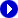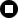# Building the Calendar

In this section were going to start building the calendar that will display a single month, which we will expand upon in the second part of this tutorial. Create a new PHP page in Dreamweaver MX, and save it as single_month.php.

## Displaying the current month

There are two steps to creating the calendar, creating the HTML and then making it dynamic.  It always helps to create a mock up in plain HTML of how you want the end result to look, and then you can create your dynamic PHP that creates the actual HTML. Using this method means its much more likely that your code creates valid HTML, as there's less chance of forgetting closing tags etc.

### Creating the HTML

To create the calendar we'll use two tables. The first table is the outer frame and has 2 rows and 1 column, in the example below the table is 280 pixels wide. In the bottom row, there is another table with 6 rows and 7 columns, each column being 40 pixels wide (40 x 7 = 280 pixels)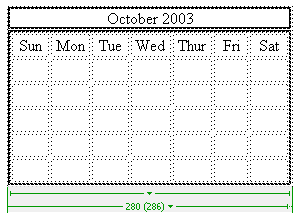Figure 1 – Example HTML layout

Now that we have the HTML structure created, we can use PHP to automatically generate the HTML code needed, and to fill in the date dynamically.

### Creating the PHP

The first step to creating the PHP is to create the basic table layout. To do this, an easy way is to copy and paste from your HTML calendar code, and convert it to be outputted through PHP. Because of the amount of code that will be dynamic in this example, it's easier to generate the whole calendar using PHP, rather than inserting PHP into the HTML.

At the moment, we just need the basic layout, so we can leave out the rows that will contain dates as we'll add them next. The code below is shown as an example:

<?php
echo "<table width='280' border='1'>\n";
echo "<tr>\n";
echo "<td align='center'>October 2003</td>\n";
echo "</tr>\n";
echo "<tr>\n";
echo "<td>\n";
echo "<table width='280'  border='0' cellspacing='2' cellpadding='2'>\n";
echo "<tr align='center'>\n";
echo "<td width='40'>Sun</td>\n";
echo "<td width='40'>Mon</td>\n";
echo "<td width='40'>Tue</td>\n";
echo "<td width='40'>Wed</td>\n";
echo "<td width='40'>Thur</td>\n";
echo "<td width='40'>Fri</td>\n";
echo "<td width='40'>Sat</td>\n";
echo "</tr>\n";
echo "</table>\n";
echo "</td>\n";
echo "</tr>\n";
echo "</table>\n";
?>

Code Block 1 – Creating the basic framework using PHP

When you're creating a table like this using PHP, it makes it much easier to see what's going on if you tabulate the code as shown above to preserve the structure. This makes it much easier to spot if you have missed a closing HTML tag e.g. </tr> and makes debugging much easier.

When this code is viewed in a browser, the following output is displayed.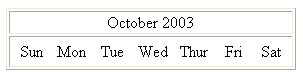Figure 2 – Basic Layout

Not very exciting at the moment but we have our basic layout in place. We can now expand on this to add the dates that need to be displayed.

The first job we have is to work out which day of the week the first day of the month falls on. We can do this with the following block of PHP, which is placed before our previous code.

\$month = date("n");
\$year = date("Y");
\$firstDay = mktime(0,1,0,\$month,1,\$year);
\$daysInMonth = date("t",\$firstDay);
\$firstDay = date("w",\$firstDay);

Code Block 2 – Calculating the first day of the month and the number of days in the month

First, we use the token "n" which places the current month number in \$month, and the token "Y" which represents the year using 4 figures and places it in \$year. Next, we need to create a timestamp which corresponds to the first day of the month e.g. \$month/1/\$year. To do this we use the PHP mktime() command which has the following format:

\$timeStamp = mktime(hour,minute,second,month,day,year);

So, to create a timestamp representing \$month/1/\$year we use mktime(0,1,0,\$month,1,\$year) which represents 00:01:00 on \$month/1/\$year. Now that we have a timestamp, we can use it with the date() parameter to see which day of the week it falls upon, which is placed in \$firstDay.

Now that we know what day of the week the first of the month falls on, we can put the first in the correct cell of the table.

Finally, we use the date() command again, with the token "t" to find out the number of days in the month. Now we know these two important bits of information we can complete the table containing the dates.

We'll now add the code to create the rest of the table and fill in the dates. To keep things clear, the code for the whole page is shown below. We'll then look at the code parts in sections.

<?php
\$month = date("n");
\$year = date("Y");
\$firstDay = mktime(0,1,0,\$month,1,\$year);
\$daysInMonth = date("t",\$firstDay);
\$firstDay = date("w",\$firstDay);
echo "<table width='280'  border='1'>\n";
echo "<tr>\n";
echo "<td align='center'>" . date("F Y") . "</td>\n";
echo "</tr>\n";
echo "<tr>\n";
echo "<td>\n";
echo "<table width='280'  border='0' cellspacing='2' cellpadding='2'>\n";
echo "<tr align='center'>\n";
echo "<td width='40'>Sun</td>\n";
echo "<td width='40'>Mon</td>\n";
echo "<td width='40'>Tue</td>\n";
echo "<td width='40'>Wed</td>\n";
echo "<td width='40'>Thu</td>\n";
echo "<td width='40'>Fri</td>\n";
echo "<td width='40'>Sat</td>\n";
echo "</tr>\n";
# Calculate number of rows
\$totalCells = \$firstDay + \$daysInMonth;
if(\$totalCells < 36){
\$rowNumber = 5;
} else {
\$rowNumber = 6;
}
\$dayNumber = 1;
# Create Rows
for(\$currentRow=1; \$currentRow <= \$rowNumber; \$currentRow++){
if(\$currentRow == 1){
# Create First Row
echo "<tr align='center'>\n";
for(\$currentCell  = 0; \$currentCell<7; \$currentCell++){
if(\$currentCell == \$firstDay){
# First Day of the Month
echo "<td width='40'>" . \$dayNumber . "</td>\n";
\$dayNumber++;
} else {
if(\$dayNumber > 1){
# First Day Passed so output Date
echo "<td width='40'>" . \$dayNumber . "</td>\n";
\$dayNumber++;
} else {
# First Day Not Reached so display blank cell
echo "<td width='40'>&nbsp;</td>\n";
}
}
}
echo "</tr>\n";
} else {
# Create Remaining Rows
echo "<tr align='center'>\n";
for(\$currentCell = 0; \$currentCell < 7; \$currentCell++){
if(\$dayNumber > \$daysInMonth){
# Days in month exceeded so display blank cell
echo "<td width='40'>&nbsp;</td>\n";
} else {
echo "<td width='40'>" . \$dayNumber . "</td>\n";
\$dayNumber++;
}
}
echo "</tr>\n";
}
}
echo "</table>\n";
echo "</td>\n";
echo "</tr>\n";
echo "</table>\n";
?>

Code Block 3 - Complete Code for displaying a Calendar Month

At the moment, we have the basic table constructed, and a row containing the days of the week. We need to now fill in the rest of the rows with the appropriate dates. The first job is to work out the number of rows that our table will need, which uses the code block shown below:

# Calculate number of rows
\$totalCells = \$firstDay + \$daysInMonth;
if(\$totalCells < 36){
\$rowNumber = 5;
} else {
\$rowNumber = 6;
}
\$dayNumber = 1;

Code to calculate the number of rows needed to display the calendar dates

The number of rows needed depends on two things. First, is the day of the month (if the first day of the month falls on a Saturday, there can be only one date in the first row), and second the number of days in the current month. Either way we will need either 5 or 6 rows, we just need to work out which.

To do this we first add the weekday number (0-6) to the number of days in the month. If the result is less than 36 we can fit the dates into 5 rows (7 * 5 = 35), otherwise we will require 6 rows to show the data. The variable \$rowNumber is set to the appropriate number of rows.

Finally, we create a new variable \$dayNumber which will hold the current date that we're working on.

Next we have part of the structure shown below:

for(\$currentRow=1; \$currentRow <= \$rowNumber; \$currentRow++){
if(\$currentRow == 1){
# Code removed for clarity
} else {
# Code removed for clarity
}
}

Basic structure of the code to generate the calendar roads

This has the code removed from the inner section for clarity. What we are doing is setting up a for loop which will run once for each row of the table that we are creating. Inside that we check if we are working on the first row of the date section or one of the other rows.

First we'll look at the section of code that creates the first row, this block is shown below:

if(\$currentRow == 1){
# Create First Row
echo "<tr align='center'>\n";
for(\$currentCell = 0; \$currentCell<7; \$currentCell++){
if(\$currentCell == \$firstDay){
# First Day of the Month
echo "<td width='40'>" . \$dayNumber . "</td>\n";
\$dayNumber++;
} else {
if(\$dayNumber > 1){
# First Day Passed so output Date
echo "<td width='40'>" . \$dayNumber . "</td>\n";
\$dayNumber++;
} else {
# First Day Not Reached so display blank cell
echo "<td width='40'>&nbsp;</td>\n";
}
}
}
echo "</tr>\n";
} else {

Code block to generate the first date row

We have to create different code to create the first row so that we can take into account which day of the week that the month started on.

First we output the HTML <tr> tag for the first row of the table.

We then have a for loop which will run 7 times, once for each column in the row. We then work through each cell, checking whether the current cell is equal to the position of the first day of the month. If it is, then we output 1 and increment \$dayNumber by 1 so that it contains the next date.

If the position of the current cell does not equal the \$currentCell value we can have one of two situations. The first is that we haven't reached the first day of the month position yet, in which case \$dayNumber will still be 1, and we output a blank cell. If we have gone past the first day of the month position then we can simply output a cell containing the current day number.

We then output a closing HTML </tr> tag to close the first row.

Finally, we can output the rest of the rows in the table which we do with the rest of the code, repeated below:

} else {
# Create Remaining Rows
echo "<tr align='center'>\n";
for(\$currentCell = 0; \$currentCell < 7; \$currentCell++){
if(\$dayNumber > \$daysInMonth){
# Days in month exceeded so display blank cell
echo "<td width='40'>&nbsp;</td>\n";
} else {
echo "<td width='40'>" . \$dayNumber . "</td>\n";
\$dayNumber++;
}
}
echo "</tr>\n";
}
}

Code to display the other date rows

This section of code is simpler. We just have to keep outputting the date until we have reached the figure indicating the number of days in the month, and then we just output blank cells until the table is completed.

As before, we first output an opening HTML <tr> tag. We then have a for loop which runs 7 times, once for each column in the row.

If we have exceeded the number of days in the month, which is stored in \$daysInMonth then we output a blank cell. If the day number hasn't exceeded the number of days in the month then we output the day number, and then increment the day number by 1.

Finally, we close the row with an HTML </tr> tag to make sure the HTML is valid.

Formatting Tip

You may have noticed that throughout this tutorial many HTML tags have had the character "\n" at the end, as shown in the example code below.

echo "<td width='40'>Sun</td>\n";
echo "<td width='40'>Mon</td>\n";
echo "<td width='40'>Tue</td>\n";
echo "<td width='40'>Wed</td>\n";
echo "<td width='40'>Thu</td>\n";
echo "<td width='40'>Fri</td>\n";
echo "<td width='40'>Sat</td>\n";

When you use PHP to output HTML, if you view the source in a browser, the original formatting is lost (and we're only talking about viewing the source here rather than the actual web display). Without the new line character (\n) the output above would look like that below:

<td width='40'>Sun</td><td width='40'>Mon</td><td width='40'>Tue</td><td width='40'>Wed</td><td width='40'>Thu</td><td width='40'>Fri</td><td width='40'>Sat</td>

This makes it difficult to spot errors in the HTML generated whilst you're debugging. By appending the new line character to the end of the line, the formatting stays as intended in the browser's view source mode, and is much easier to debug, and also looks more professional.

<td width='40'>Sun</td>
<td width='40'>Mon</td>
<td width='40'>Tue</td>
<td width='40'>Wed</td>
<td width='40'>Thu</td>
<td width='40'>Fri</td>
<td width='40'>Sat</td>

The Calendar Output

When the code in Code Block 3 (full code) is run, you should see something similar to the screenshot below.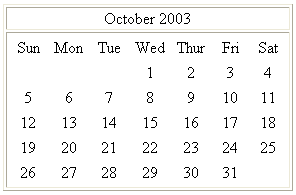Figure 3 – Example output

In the example above you can see that the first day of the month is shown under the correct day, and that the month is shown with the correct number of days.

In the next part of this tutorial, we will look at styling the calendar using CSS, and making more advanced calendars that can interact with a database.### Gareth Downes-PowellGareth has a range of skills, covering many computer and internet related subjects. He is proficient in many different languages including ASP and PHP, and is responsible for the setup and maintenance of both Windows and Linux servers on a daily basis.

In his daily web development work he uses the complete range of Macromedia software, including Dreamweaver MX, Flash MX, Fireworks MX and Director to build a number of websites and applications. Gareth has a close relationship with Macromedia, and as a member of Team Macromedia Dreamweaver, he has worked closely in the development of Dreamweaver, and was a beta tester for Dreamweaver MX.

On a daily basis he provides support for users in the Macromedia forums, answering questions and providing help on a range of different web related subjects. He has also written a number of free and commercial extensions for Dreamweaver MX, to further extend its capabilities using its native JavaScript API’s or C++.

As a web host, Gareth has worked with a range of different servers and operating systems, with the Linux OS as his personal favourite. Most of his development work is done using a combination of Linux, Apache and MySQL and he has written extensively about setting up this type of system, and also running Apache and MySQL under Windows.

See All Postings From Gareth Downes-Powell >>

## Reviews#### Revised Version ?

June 6, 2008 by John Ashton

Having used this great tutorial some years ago when it first appeared I was keen to learn about the revised version and the methods used to interact with a database. So, I purchased the Tut, downloaded then read it only to find that this is the same as I previously had.

So how do you intend to provide what was advertised, "Creating a simple calendar in PHP (updated) ???

Not happy guys !!